Question

# This figure shows a sinusoidal wave that is traveling from left to right, in the +x-direction....

This figure shows a sinusoidal wave that is traveling from left to right, in the +x-direction. Assume that it is described by a frequency of 61.0 cycles per second, or hertz (Hz). 4.91 cm3.10 cm A sinusoidal wave lies on an unlabeled coordinate system. One of the wave's maxima lies on the vertical axis. The horizontal distance from the first maximum to the first minimum is labeled 3.10 cm and the vertical distance between a maximum and a minimum is labeled 4.91 cm. (a) What is the wave's amplitude (in cm)? (No Response) cm (b) What is the wavelength (in cm)? (No Response) cm (c) Calculate the wave's period (in s). (No Response) s (d) Compute the speed of this wave (in m/s)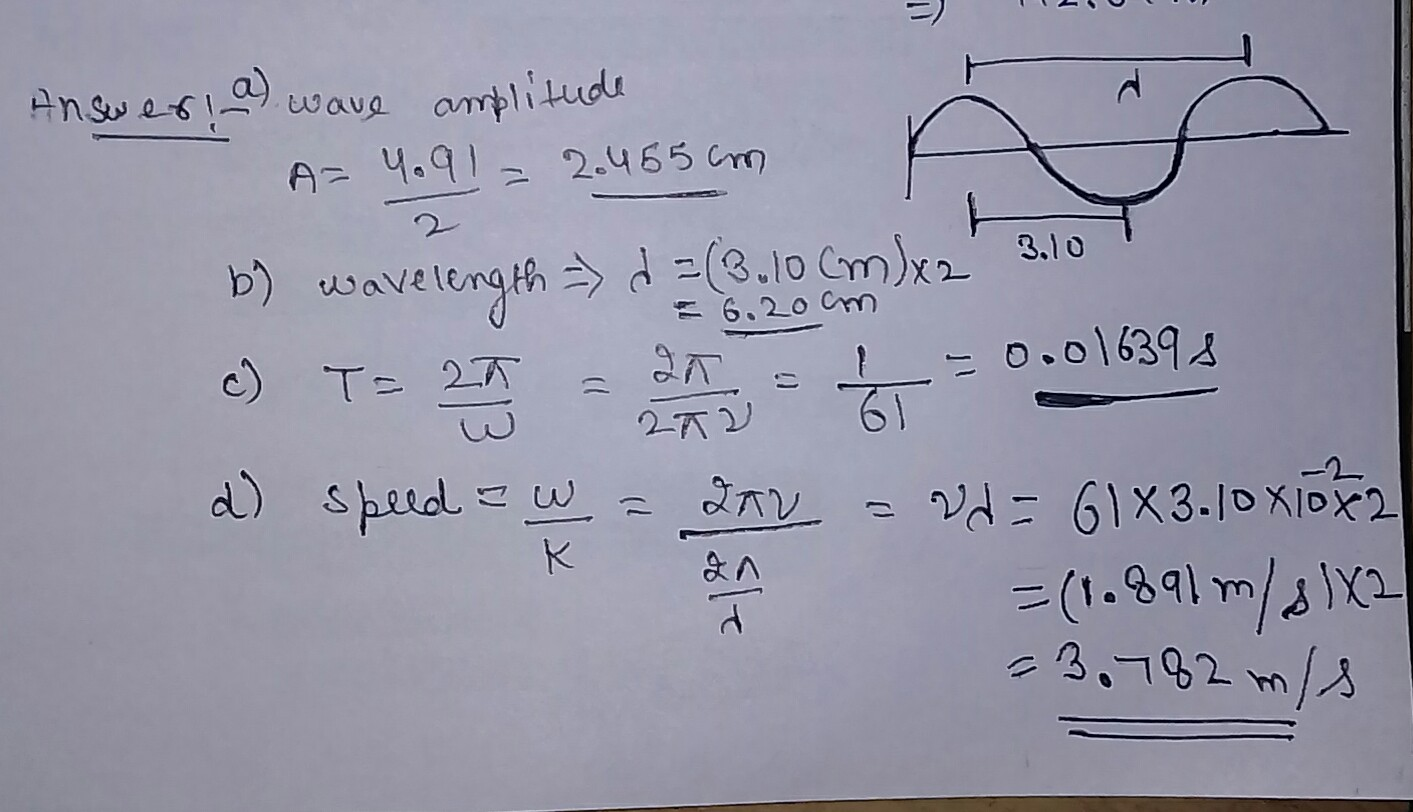wavelength is the distance between the two consecutive maxima or minima.

#### Earn Coins

Coins can be redeemed for fabulous gifts.

Similar Homework Help Questions
• ### The sinusoidal wave shown in the figure below is traveling in the positive x-direction and has...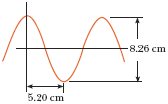The sinusoidal wave shown in the figure below is traveling in the positive x-direction and has a frequency of 20.6 Hz. (a) Find the amplitude. cm? (b) Find the wavelength. cm? (c) Find the period. s? (d) Find the speed of the wave. m/s?

• ### A sinusoidal wave traveling in the positive X direction has an amplitude of 10.0 cm, a...

A sinusoidal wave traveling in the positive X direction has an amplitude of 10.0 cm, a wavelength of 25.0 cm, and a frequency of 5.00 Hz. c) wave function of time

• ### Sinusoidal transverse wave

A sinusoidal transverse wave travels along a long stretch string. The amplitude of this wave is 0.0839 m, its frequency is 2.77 hz, and its wavelength is 1.79m.What is the transverse distance between a maximum and a minimum of the wave? (m)How much time is required for 79.7 cycles of the wave to pass a stationary obsever? (s)Viewing the whole wave at any instant, how many cycles are there in a 34.5 m length of string?(cycles)

• ### A sinusoidal wave traveling in the positive X direction has an amplitude of 0.10 m cm,...

A sinusoidal wave traveling in the positive X direction has an amplitude of 0.10 m cm, a wavelength of 0.25 m, and a frequency of 50 Hz. Find the followings: For each question you Must show the symbol, formula, calculations, result, and unit Period.    Angular frequency. wave number. Speed of the wave (phase velocity) Maximum speed of vibration (transverse speed) of the source of the wave Maximum acceleration of the vibration (transverse acceleration) The equation for the moving wave.

• ### A sinusoidal transverse wave travels along a long, stretched string. The amplitude of this wave is...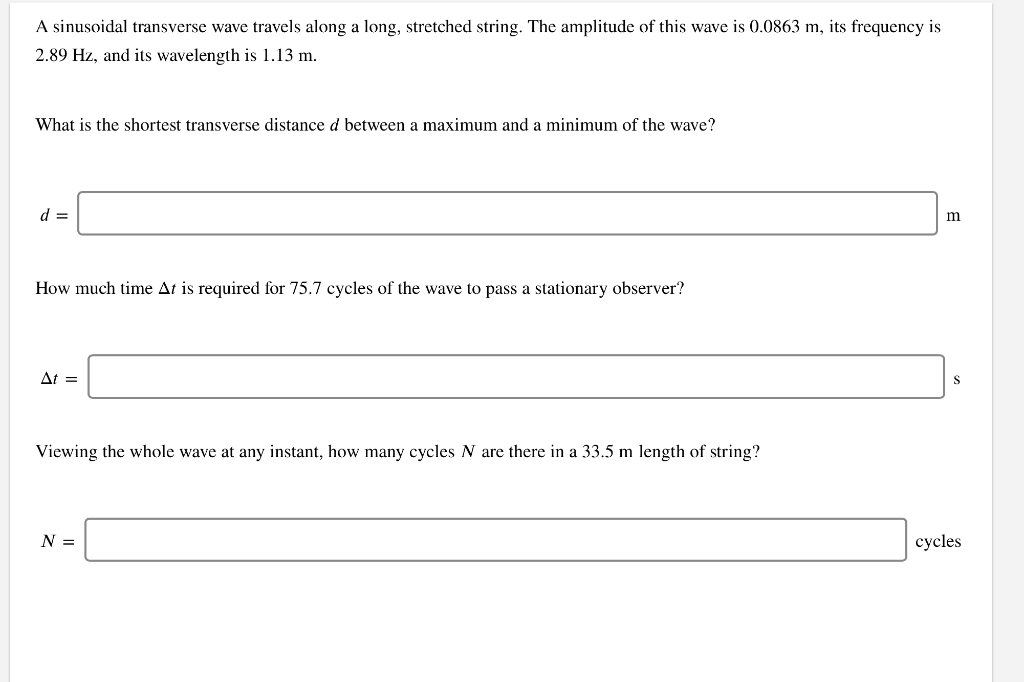A sinusoidal transverse wave travels along a long, stretched string. The amplitude of this wave is 0.0863 m, its frequency is 2.89 Hz, and its wavelength is 1.13 m. What is the shortest transverse distance d between a maximum and a minimum of the wave? d = m How much time At is required for 75.7 cycles of the wave to pass a stationary observer? At = S Viewing the whole wave at any instant, how many cycles N are...

• ### 3) A sinusoidal wave traveling in the +x direction has a wavelength of 20 cm, a...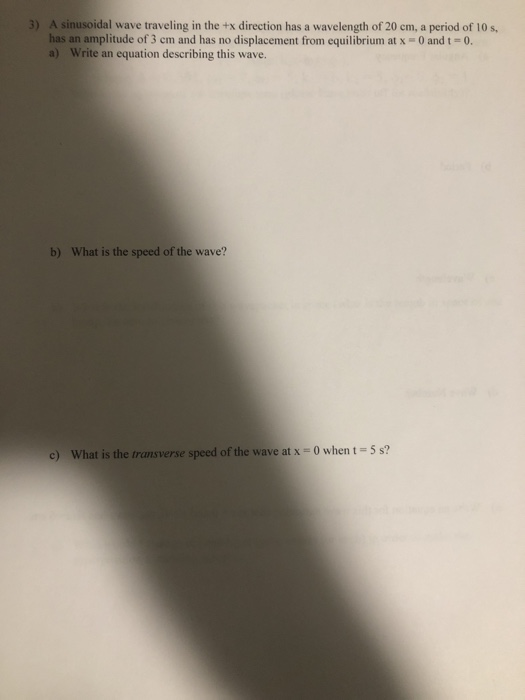3) A sinusoidal wave traveling in the +x direction has a wavelength of 20 cm, a period of 10 s. has an amplitude of 3 cm and has no displacement from equilibrium at x and t-0. a) Write an equation describing this wave. b) What is the speed of the wave? c) What is the transverse speed of the wave at x = 0 when t-5s?

• ### A sinusoidal transverse wave is traveling along a string in the negative direction of an x...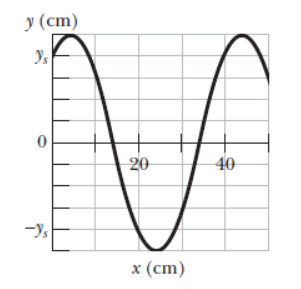A sinusoidal transverse wave is traveling along a string in the negative direction of an x axis. The figure below shows a plot of the displacement as a function of position at time t = 0. The x axis is marked in increments of 10 cm and the y axis is marked in increments of 2 cm. The string tension is 3.1 N, and its linear density is 34 g/m. (a) Find the amplitude. m (b) Find the wavelength. m...

• ### A sinusoidal transverse wave travels along a long, stretched string. The amplitude of this wave is...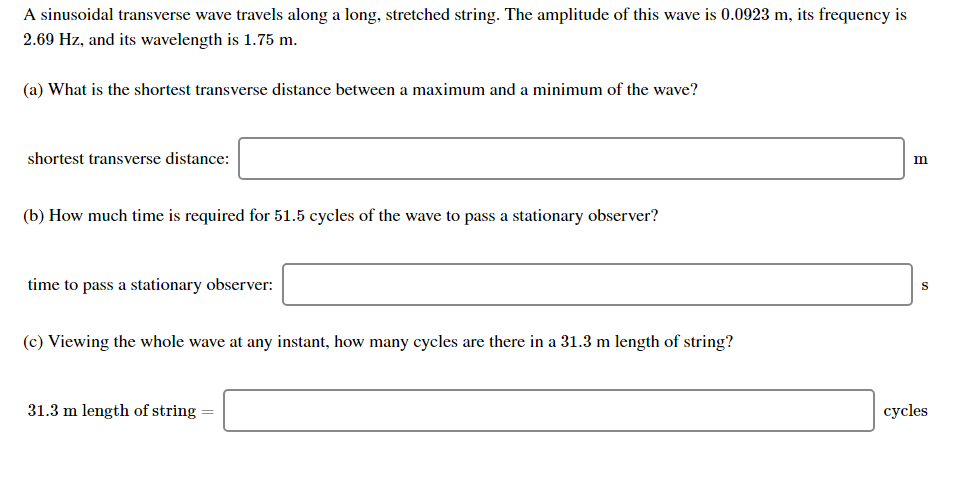A sinusoidal transverse wave travels along a long, stretched string. The amplitude of this wave is 0.0923 m, its frequency is 2.69 Hz, and its wavelength is 1.75 m. (a) What is the shortest transverse distance between a maximum and a minimum of the wave? shortest transverse distance: (b) How much time is required for 51.5 cycles of the wave to pass a stationary observer? time to pass a stationary observer: (c) Viewing the whole wave at any instant, how...

• ### A sinusoidal transverse wave travels along a long, stretched string. The amplitude of this wave is...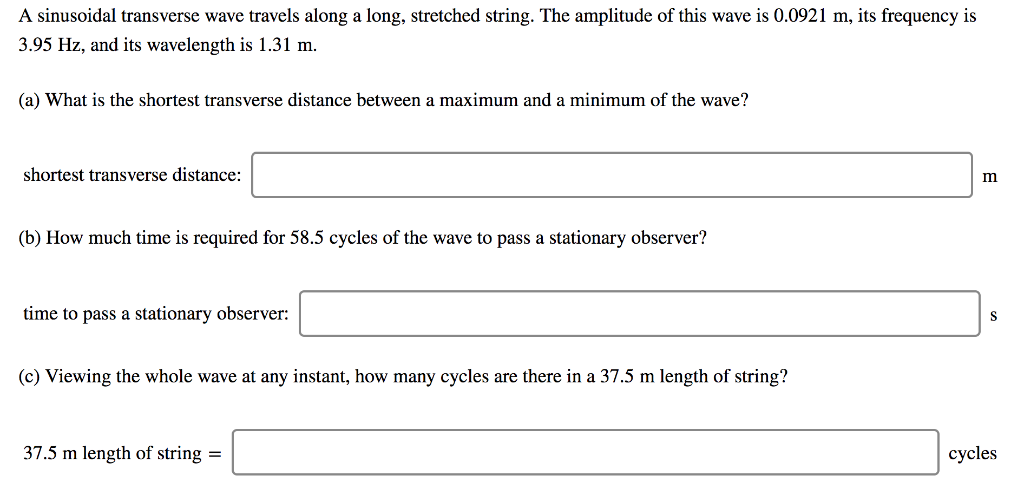A sinusoidal transverse wave travels along a long, stretched string. The amplitude of this wave is 0.0921 m, its frequency is 3.95 Hz, and its wavelength is 1.31 m. (a) What is the shortest transverse distance between a maximum and a minimum of the wave? shortest transverse distance: (b) How much time is required for 58.5 cycles of the wave to pass a stationary observer? time to pass a stationary observer: (c) Viewing the whole wave at any instant, how...

• ### sinusoidal wave is traveling

A sinusoidal transverse wave is traveling along a string in the negative direction of an x axis. The figure below shows a plot of the displacement as a function ofposition at time t = 0. The x axis is marked in increments of 25 cm and the y axis is marked in increments of 2 cm. The string tension is 2.5 N, and its lineardensity is 21 g/m.(a) Find the amplitude.m(b) Find the wavelength.m(c) Find the wave speed.m/s(d) Find the...